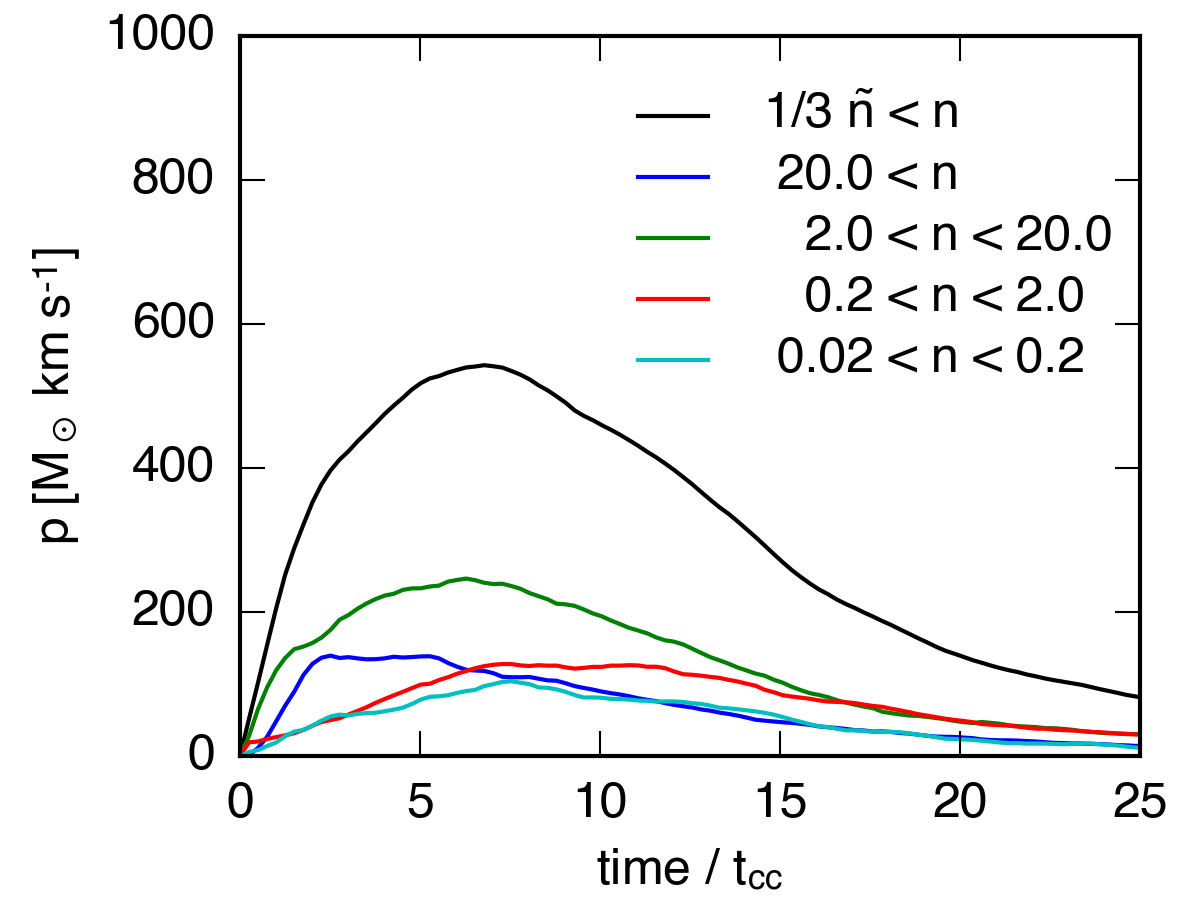# Integrated Mass and Momentum Evolution

## June 10, 2016

The following are plots of the integrated mass in different density bins for each of the 6 high resolution simulations I’ve run. On the y-axis I’ve plotted the total mass in each density bin, including the total mass above 1/3 the original median density of the cloud.

$\tilde{n} = 1$ cloud: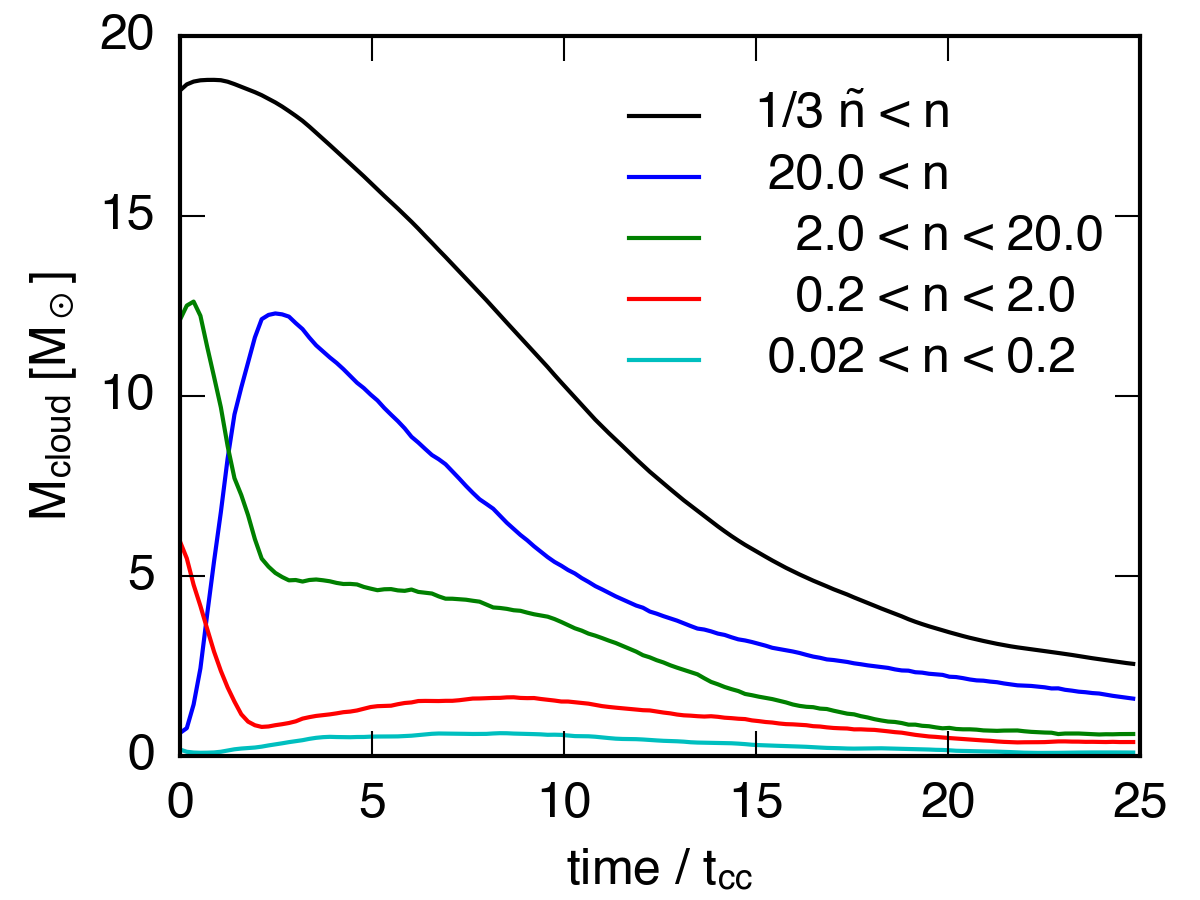$\tilde{n} = 1$ sphere: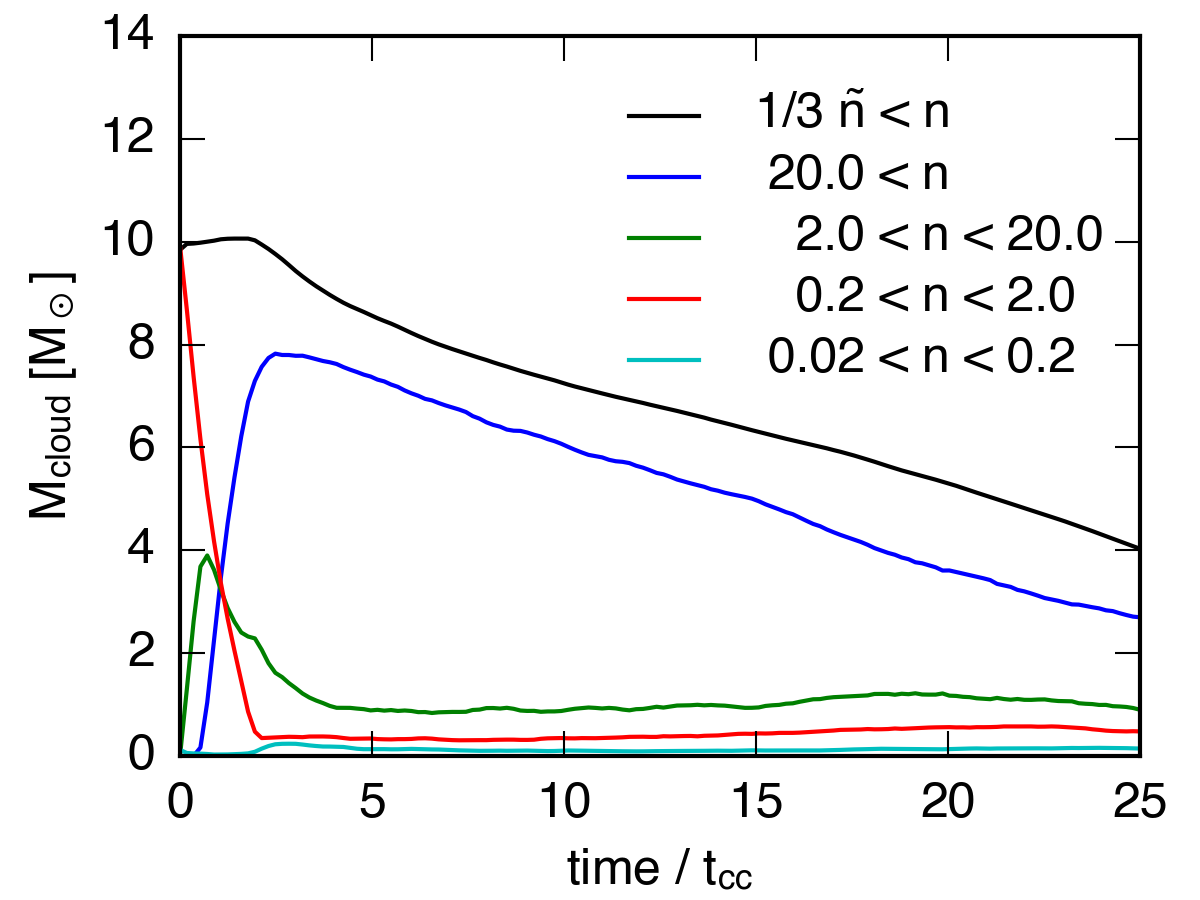$\tilde{n} = 0.5$ cloud: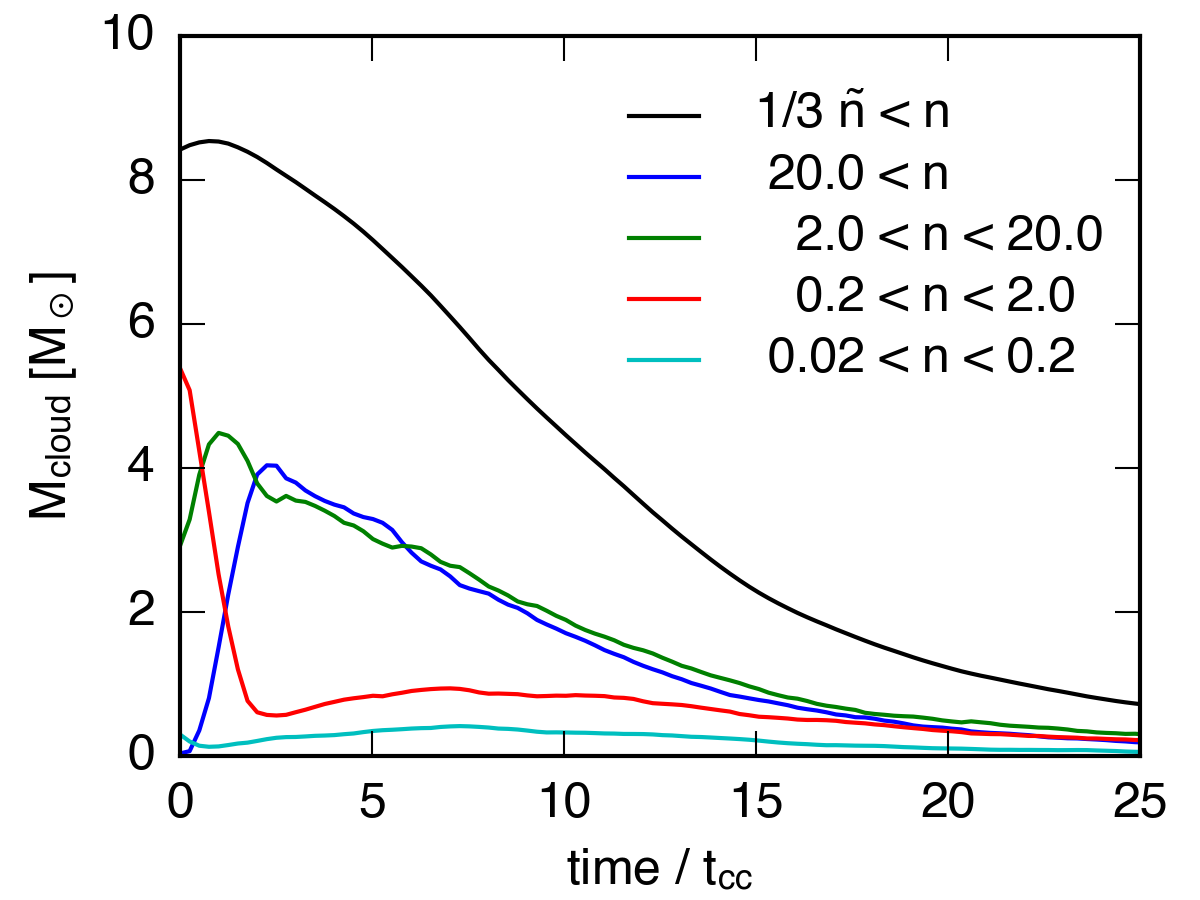$\tilde{n} = 0.5$ sphere: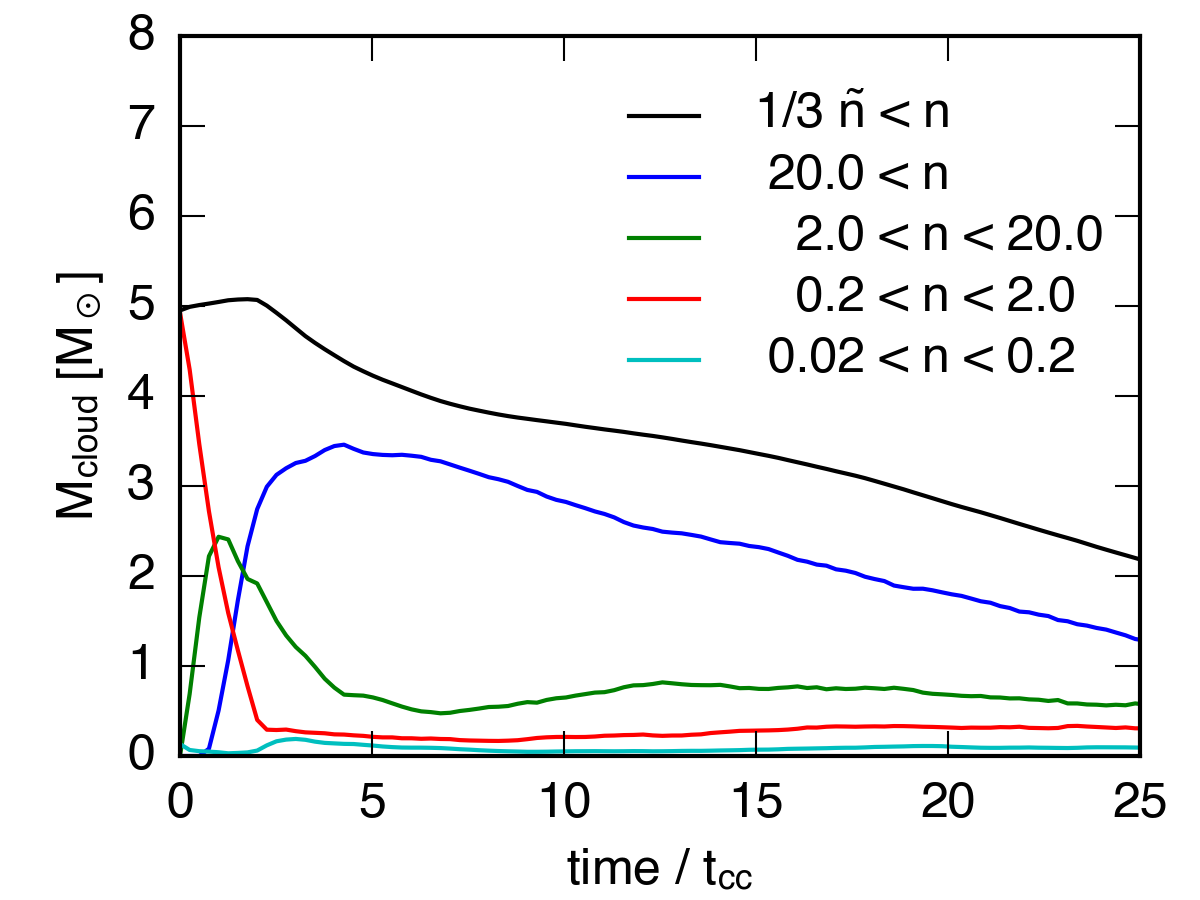$\tilde{n} = 0.1$ cloud: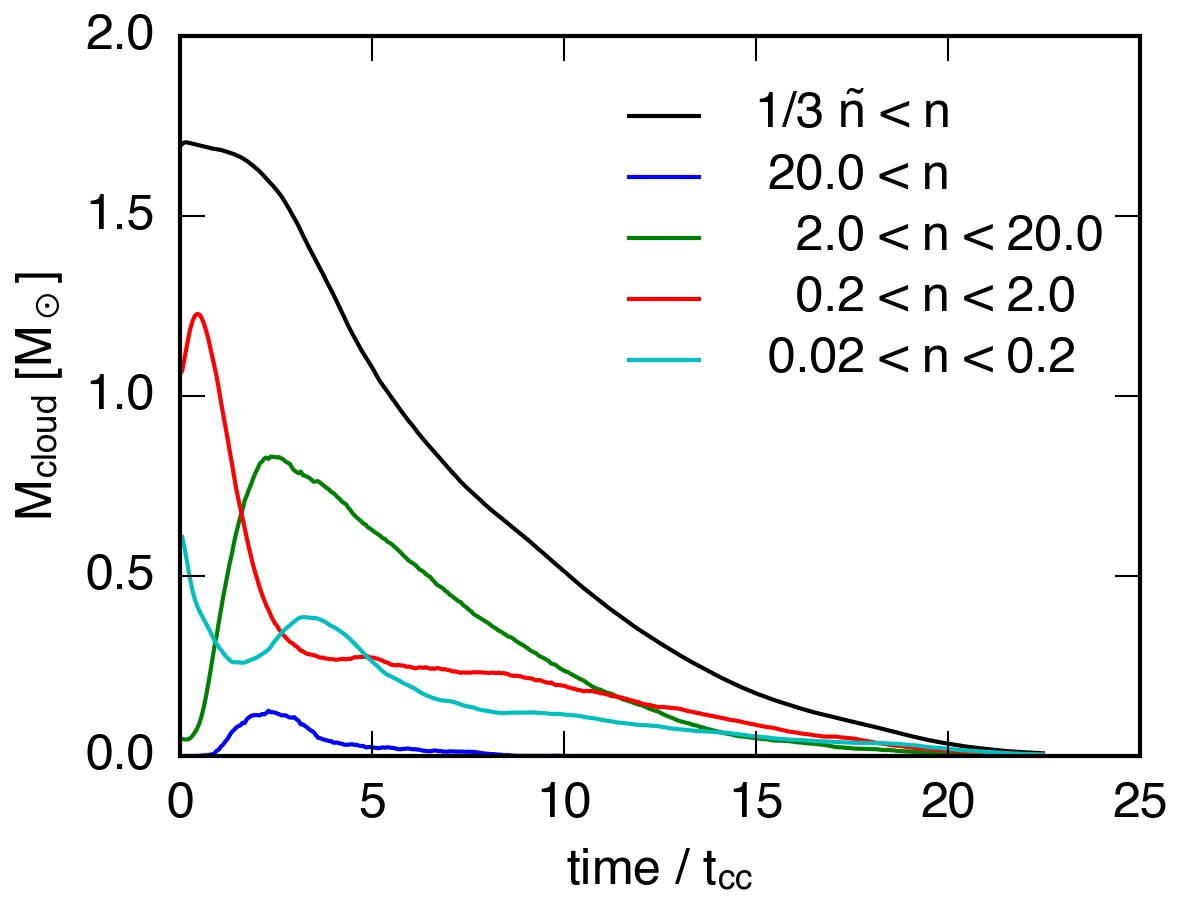$\tilde{n} = 0.1$ sphere: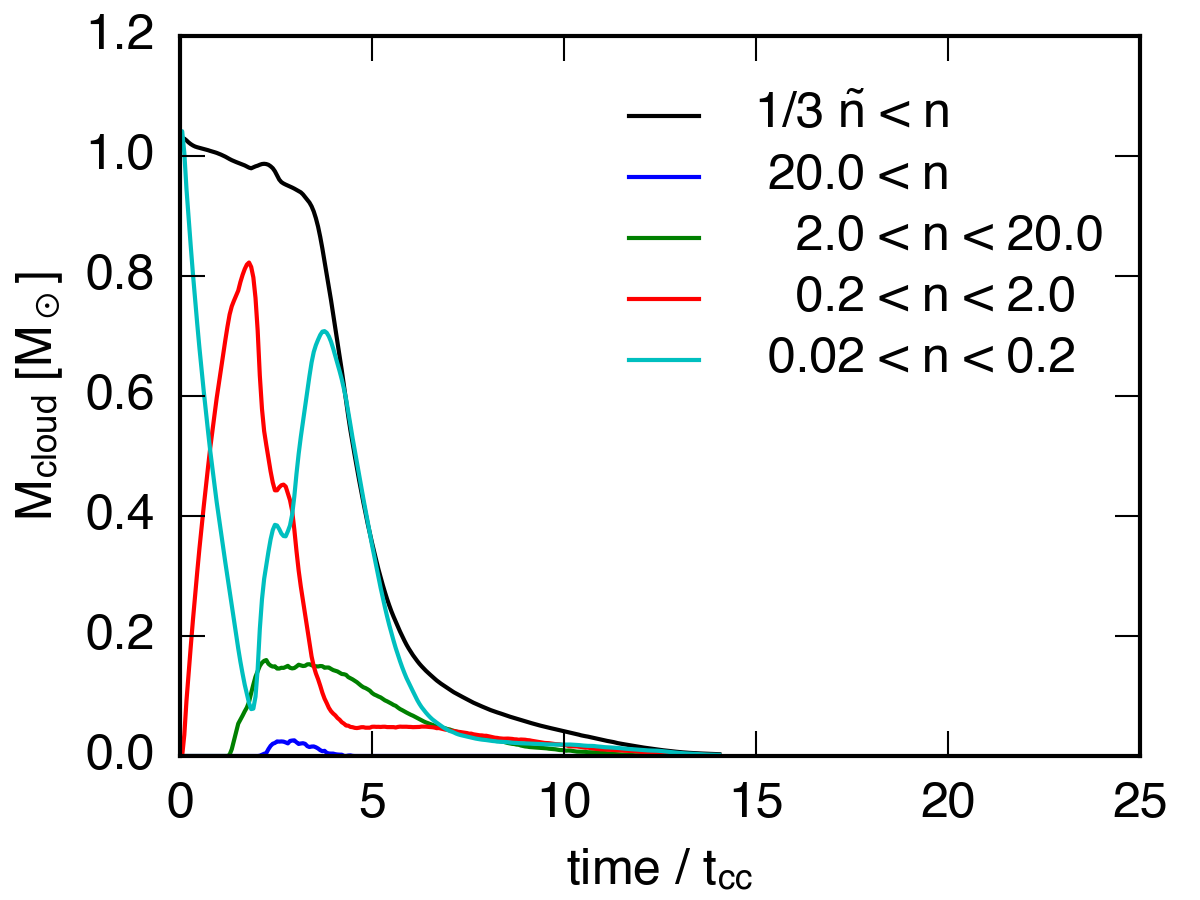I’ve also plotted the integrated momentum for each of the 3 highest surface density simulations, using the same density bins.

$\tilde{n} = 1$ cloud: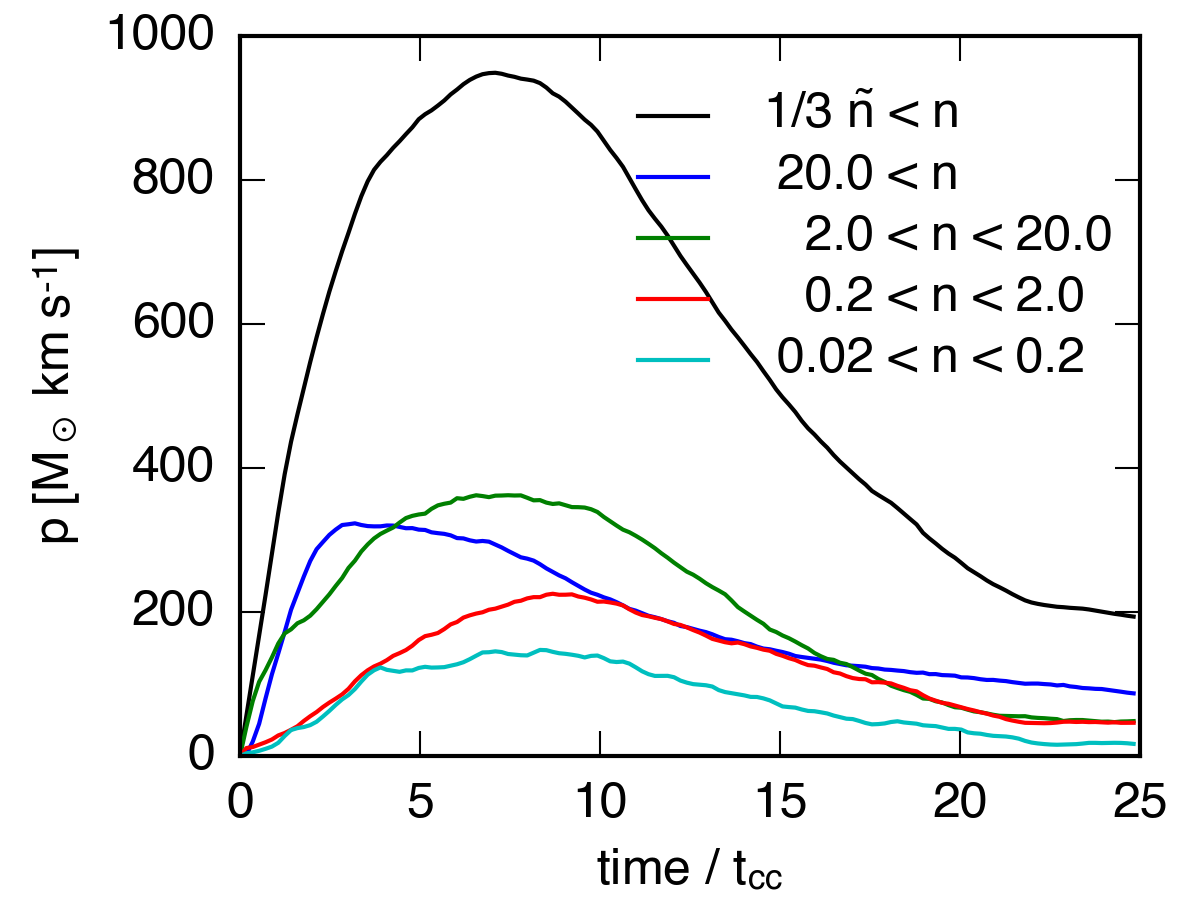$\tilde{n} = 1$ sphere: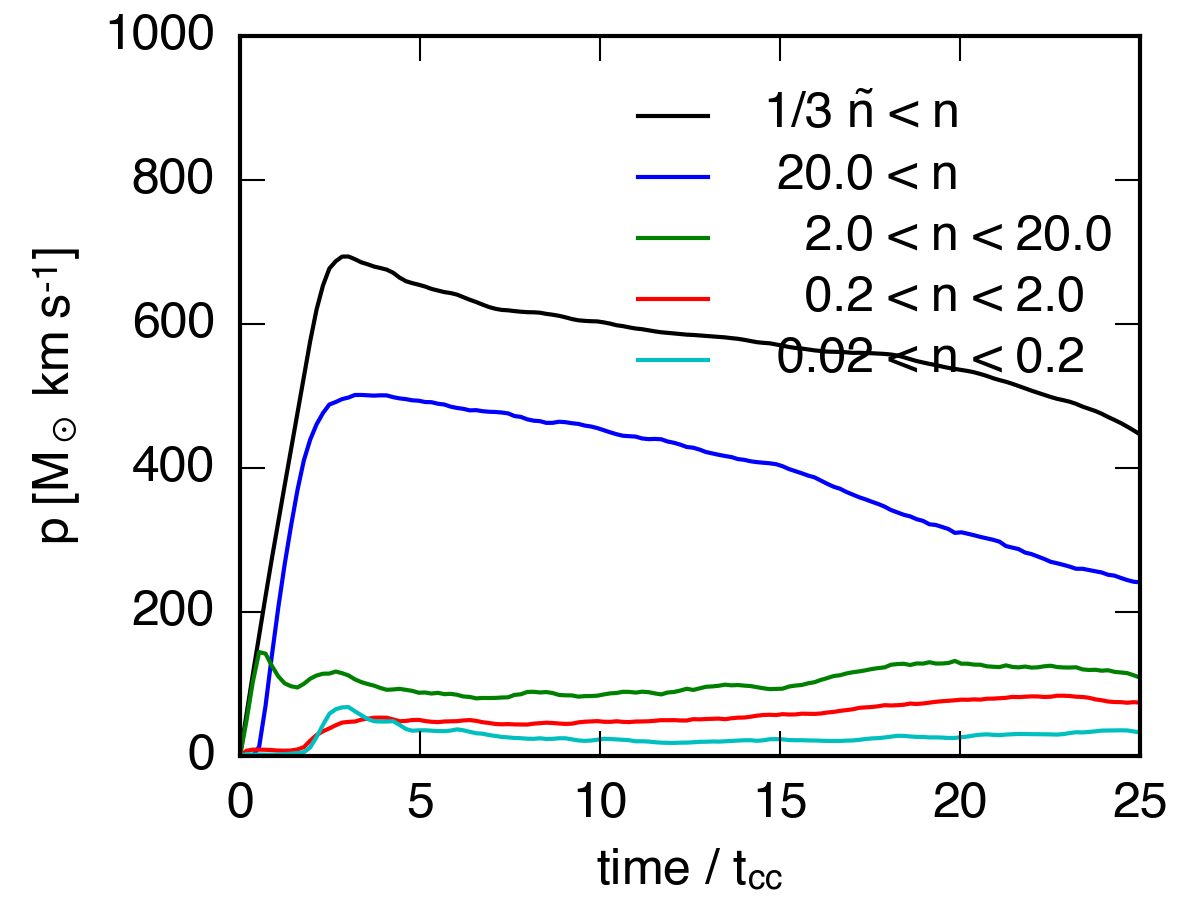$\tilde{n} = 0.5$ cloud: